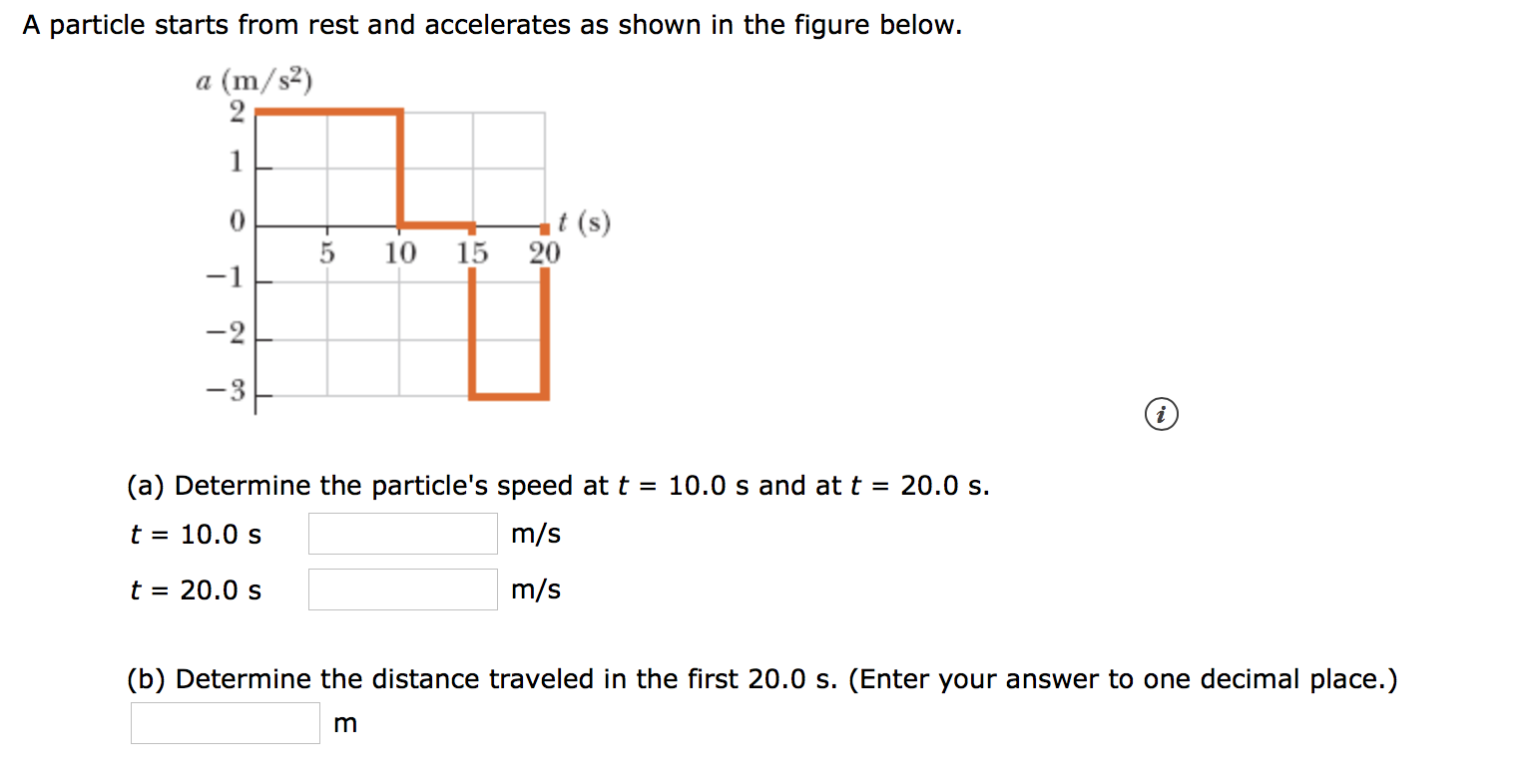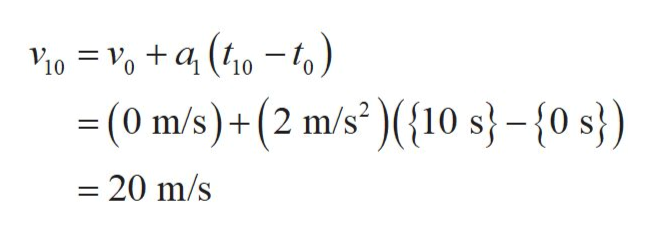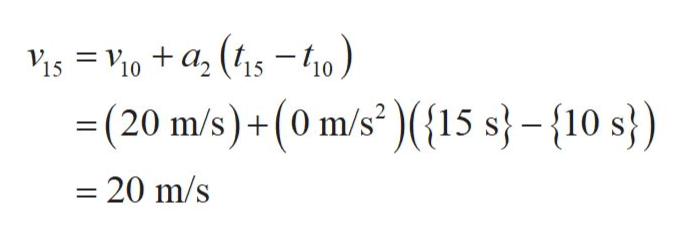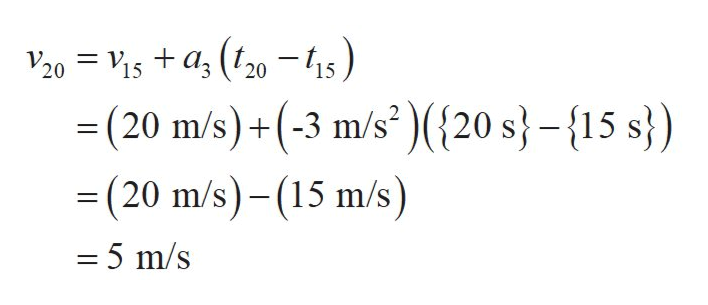A particle starts from rest and accelerates as shown in the figure below.(m/s2)a1t (s)15 20510-1-2(a) Determine the particle's speed at t = 10.0 s and at t = 20.0 s.10.0 sm/stt 20.0 sm/s(b) Determine the distance traveled in the first 20.0 s. (Enter your answer to one decimal place.)m

Questionhelp_outlineImage TranscriptioncloseA particle starts from rest and accelerates as shown in the figure below. (m/s2) a 1 t (s) 15 20 5 10 -1 -2 (a) Determine the particle's speed at t = 10.0 s and at t = 20.0 s. 10.0 s m/s t t 20.0 s m/s (b) Determine the distance traveled in the first 20.0 s. (Enter your answer to one decimal place.) m fullscreen
Step 1

Between t = 0 s and t = 10 s, the particle’s acceleration is 2 m/s2.

Use kinematics’ first equation to find the velocity v10 of the particle at t =10.0 s as,help_outlineImage Transcriptionclose10 10 (0 m/s)+(2 m/s(10 s}-{0 s}) - 20 m/s fullscreen
Step 2

Here, v0 is the particle’s velocity at t = 0 s and a1 is the particle’s acceleration between 0 s and 10 s.

Between t = 10 s and t = 15 s, the particle’s acceleration is 0 m/s2.

Use kinematics’ first equation to find the particle’s velocity v15 at t =15.0 s as,help_outlineImage Transcriptionclose= V. 10 15 10 =(20 m/s)+(0 m/s(15 s-10 s) = 20 m/s fullscreen
Step 3

Here, a2 is the particle’s acceleration between 10 s and 15 s.

Between t = 15 s and t = 20 s, the particle’s acceleration is -3 m/s2.

Use kinema...help_outlineImage TranscriptioncloseV20 is +a (20-4s) (20 m/s)+(-3 m/s) ({20 s}-{15 s}) =(20 m/s)-(15 m/s) 15 15 = 5 m/s fullscreen

Want to see the full answer?

See Solution

Want to see this answer and more?

Our solutions are written by experts, many with advanced degrees, and available 24/7

See Solution
Tagged in

Kinematics